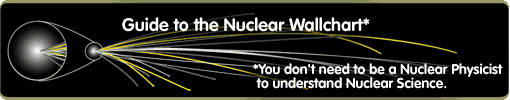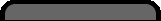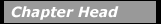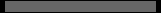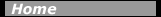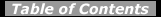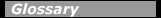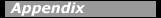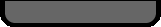## LinearThe radio-frequency (RF) linear accelerator avoids these problems by the repeated acceleration of ions through relatively small potential differences. In a linear accelerator, an ion is injected into an accelerating tube containing a number of electrodes. A high-frequency alternating voltage from an oscillator is applied between groups of electrodes. An ion traveling down the tube will be accelerated in the gap between the electrodes if the voltage is in the proper phase. The distance between electrodes increases along the length of the tube so that the particle stays in phase with the voltage.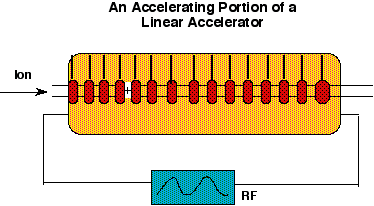Fig. 11-3. Side view of a linear accelerator. (This figure does not show the increasing spacing between cavities as discussed in the text.)

The first linear accelerator was built in 1928 by R. Wideroe, accelerating positive ions to about 50 keV. Intensive work on linear accelerators was carried out in many laboratories in the early 1930s. The linear accelerator did not receive much further attention until after World War II, when the availability of high-power microwave oscillators made possible acceleration to high energies in relatively small linear accelerators. Since that time, a sizable number of linear accelerators, also called linacs, have come into operation, both for electron and proton acceleration, as well as several heavy-ion linacs. SLAC, a 3-km electron linac at Stanford University, is the longest linac presently operating. It accelerates electrons and positrons to energies of 50 GeV.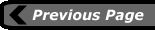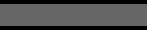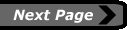last updated: August 9, 2000 webmaster